# Circles-Important Questions

Class X Math
HOTs for Facts that Matter
1.   In the following figure, two circle touch each other externally at C. Prove that the common tangent at C bisects the other two common tangents.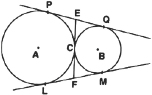2.   In the figure, if Ab = AC, prove that BE = CE.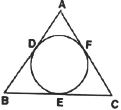3.   A point P is 13 cm from the centre of the circle. The length of the tangent drawn from P to the circle is 12 cm. Find the radius of the circle.
4.   A circle is touching the side BC of ��ABC at P and touching AB and AC produced at Q and R respectively.
Prove that: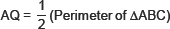5.   The incircle of ��ABC touches the sides BC, CA and AB at D, E and F respectively.
Show that:
AF+ BD + CD = AE + BF + CE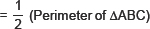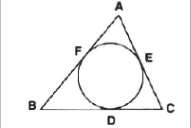6.   Show that the tangents drawn at the end points of a diameter of a circle are parallel.
7.   In the figure PQ is a chord of a circle and PT is the tangent at P such that
∠QPT = 60°, Find ∠PRQ.
8.   PQ is a chord of length 8 cm of a circle of radius 5 cm. The tangents at P and Q intersect at a point T. Find the length of TP.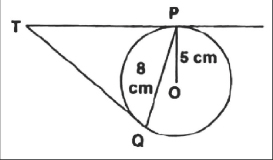9.   In the figure, two circles with centres A and B and radii 5 cm and 3 cm touching each other internally. If the perpendicular bisector of segment AB, meets the bigger circle at P and Q, find the length of PQ.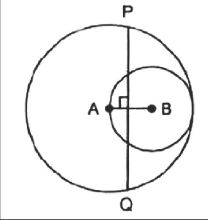10.   Two tangents making an angle of 120� with each other, are drawn to a circle of radius 6 cm. Show that the length of each tangent is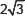cm.